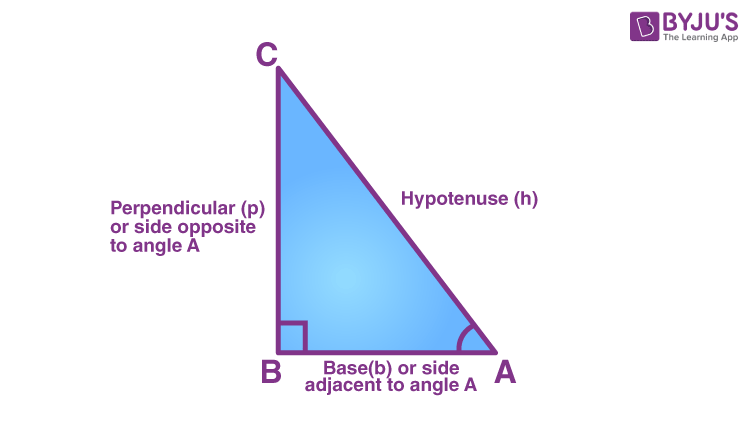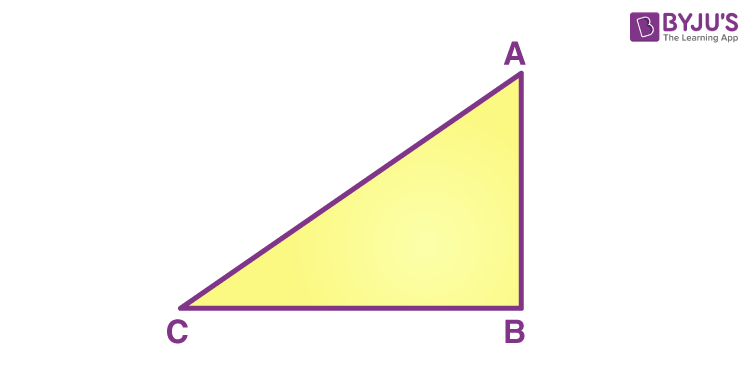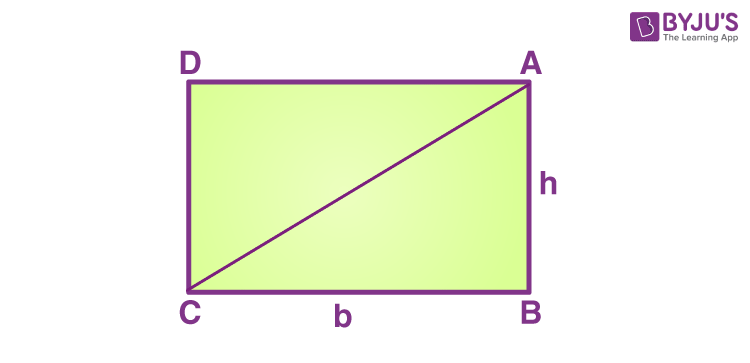# Right Triangle Formula

The right triangle formula includes the formulas of the area of a right triangle, along with its perimeter and length of the hypotenuse formula.

In geometry, you come across different types of figures, the properties of which, set them apart from one another. One common figure among them is a triangle. A triangle is a closed figure, a polygon, with three sides. It has 3 vertices and its 3 sides enclose 3 interior angles of the triangle. The sum of the three interior angles in a triangle is always 180 degrees.

The most common types of triangles that we study are equilateral, isosceles, scalene and right-angled triangles. In this section, we will talk about the right triangle formula, also called the right-angled triangle formulas.

## Right Triangle

A right triangle is the one in which the measure of any one of the interior angles is 90 degrees. It is to be noted here that since the sum of interior angles in a triangle is 180 degrees, only 1 of the 3 angles can be a right angle.

If the other two angles are equal, that is 45 degrees each, the triangle is called an isosceles right-angled triangle. However, if the other two angles are unequal, it is a scalene right-angled triangle.

The most common application of right-angled triangles can be found in trigonometry. In fact, the relation between its angles and sides forms the basis for trigonometry.

## Area of a right triangle – Formula

The area of a right triangle is the region covered by its boundaries or within its three sides.

The formula to find the area of a right triangle is given by:

 $$\begin{array}{l}Area~ of~ a~ right~ triangle = \frac{1}{2} bh\end{array}$$

Where b and h refer to the base and height of the triangle, respectively.

## Perimeter of a right triangle – Formula

The perimeter of a right triangle is a distance covered by its boundary or the sum of all its three sides.

The formula to find the perimeter of a triangle is given by:

 Perimeter of a right triangle = a + b + c

Where a, b and c are the measure of its three sides.

## Hypotenuse of a right triangle – Formula

A right triangle has three sides called the base, the perpendicular and the hypotenuse. The hypotenuse is the longest side of the right triangle. Pythagoras Theorem defines the relationship between the three sides of a right-angled triangle. Thus, if the measure of two of the three sides of a right triangle is given, we can use the Pythagoras Theorem to find out the third side.

 $$\begin{array}{l}Hypotenuse^{2} = Perpendicular^{2} + Base^{2}\end{array}$$In the figure given above, ∆ABC is a right-angled triangle that is right-angled at B. The side opposite to the right angle, which is the longest side, is called the hypotenuse of the triangle. In ∆ABC, AC is the hypotenuse. Angles A and C are the acute angles. We name the other two sides (apart from the hypotenuse) as the ‘base’ or ‘perpendicular’ depending on which of the two angles we take as the basis for working with the triangle.

## Derivation of Right Triangle Formula

Consider a right-angled triangle ABC which has B as 90 degrees and AC is the hypotenuse.Now we flip the triangle over its hypotenuse such that a rectangle ABCD with width h and length b is formed.You already know that area of a rectangle is given as the product of its length and width, that is, length x breadth.

Hence, area of the rectangle ABCD = b x h

As you can see, the area of the right angled triangle ABC is nothing but one-half of the area of the rectangle ABCD.

Thus,

$$\begin{array}{l}Area ~of \Delta ABC = \frac{1}{2} Area ~of~ rectangle ABCD\end{array}$$

Hence, area of a right angled triangle, given its base b and height

$$\begin{array}{l}A= \frac{1}{2} bh\end{array}$$

## Solved Examples on Right Triangle Formula

Question 1: The length of two sides of a right angled triangle is 5 cm and 8 cm. Find:

• Length of its hypotenuse
• Perimeter of the triangle
• Area of the triangle

Solution: Given,

One side a = 5cm

Other side b = 8 cm

• The length of the hypotenuse is,

Using Pythagoras theorem,

$$\begin{array}{l}Hypotenuse^{2} = Perpendicular^{2} + Base^{2}\end{array}$$

$$\begin{array}{l}c^{2} = a^{2} +b^{2}\end{array}$$

$$\begin{array}{l}c^{2} = 5^{2} +8^{2}\end{array}$$

$$\begin{array}{l}c= \sqrt{25+64}= \sqrt{89}= 9.43cm\end{array}$$

Perimeter of the right triangle = a + b + c = 5 + 8 + 9.43 = 22.43 cm

$$\begin{array}{l}Area ~of~ a~ right ~triangle = \frac{1}{2} bh\end{array}$$

Here, area of the right triangle =

$$\begin{array}{l}\frac{1}{2} (8\times5)= 20cm^{2}\end{array}$$

Question 2:  The perimeter of a right-angled triangle is 32 cm. Its height and hypotenuse measure 10 cm and 13cm respectively. Find its area.

Solution: Given,

Perimeter = 32 cm

Hypotenuse a= 13 cm

Height b= 10 cm

Third side, c=?

We know that perimeter = a+ b+ c

32 cm = 13 + 10 + c

Therefore, c = 32 – 23 = 9 cm

$$\begin{array}{l}Area = \frac{1}{2} bh = \frac{1}{2} (9\times10)= 45cm^{2}\end{array}$$

### Worksheet

1. What is the area of right triangle if base = 6 cm and height = 4 cm?
2. Find the area of right triangle if base = 15 cm and height = 10 cm.
3. Find the area of a right triangle if base = 6 cm and hypotenuse = 10 cm.
4. What is the perimeter of a triange if base = 3 in, height = 4 in and hypotenuse = 5 in?

To solve more problems on the topic and for video lessons, download BYJU’S -The Learning App.